Next: Coupled Oscillations Up: Hamiltonian Dynamics Previous: Hamilton's Equations

Exercises

1. A particle of mass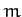is placed at the top of a smooth vertical hoop of radius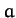. Calculate the reaction of the hoop on the particle as it slides down the hoop by means of the method of Lagrange multipliers. Find the height at which the particle falls off the hoop.
2. A uniform disk of massand radiushas a light string wrapped around its circumference with one end of the string attached to a fixed support. The disk is allowed to fall under gravity, unwinding the string as it falls. Solve the problem using the method of Lagrange multipliers. What is the tension in the string?
3. Consider two particles of masses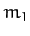and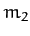. Letbe constrained to move on a circle of radiusin the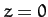plane, centered at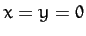. (Here,measures vertical height). Letbe constrained to move on a circle of radiusin the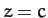plane, centered on. A light spring of spring constant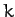and unstretched length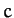is attached between the particles. Find the Lagrangian of the system. Solve the problem using Lagrange multipliers and give a physical interpretation for each multiplier.

4. Find the Hamiltonian of a particle of massconstrained to move under gravity on the inside of a sphere of radius. Use the standard spherical polar coordinatesand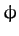as your generalized coordinates, where the axis of the coordinates points vertically downward. Find Hamilton's equations of motion for the system.
5. A particle of massis subject to a central attractive force given by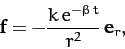whereand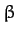are positive constants. Find the Hamiltonian of the particle. Compare the Hamiltonian to the total energy of the particle. Is the energy of the particle conserved?Next: Coupled Oscillations Up: Hamiltonian Dynamics Previous: Hamilton's Equations
Richard Fitzpatrick 2011-03-31